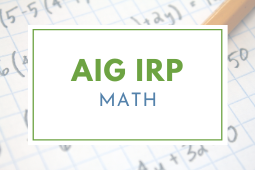Author:
Melody Casey
Subject:
English Language Arts, Mathematics
Material Type:
Lesson Plan
Level:
Lower Primary
2
Tags:
• IRPMATH
Language:
English

# Addition/Subtraction Strategies - Agree or Challenge (AIG IRP)## Overview

By now students have worked with many strategies to add and subtract numbers. Students will work in pairs. One student will think of an addition or subtraction problem and choose an efficient strategy to solve the problem. Then the student will give the problem to the other student who will solve the problem and explain his/her strategy to the partner. The problem poser will ask questions about the explanation or tell how the strategy matched the strategy he/she used. Students will discuss the benefits of a particular strategy. Then the other student will pose the next problem. The students will keep track of strategies used and try to create problems that will allow them to use more than one strategy. For the last few minutes of the activity the pairs can share out the different strategies they were able to use and explain. This lesson was developed by NCDPI as part of the Academically and/or Intellectually Gifted Instructional Resources Project. This lesson plan has been vetted at the state level for standards alignment, AIG focus, and content accuracy.

# Lesson Overview

Brief Description of Lesson/Task/Activity: By now students have worked with many strategies to add and subtract numbers. Students will work in pairs. One student will think of an addition or subtraction problem and choose an efficient strategy to solve the problem. Then the student will give the problem to the other student who will solve the problem and explain his/her strategy to the partner. The problem poser will ask questions about the explanation or tell how the strategy matched the strategy he/she used. Students will discuss the benefits of a particular strategy. Then the other student will pose the next problem. The students will keep track of strategies used and try to create problems that will allow them to use more than one strategy. For the last few minutes of the activity the pairs can share out the different strategies they were able to use and explain. Example: 1st student writes the problem 183 + 17 = ___. On her white board or paper she writes: I know that 7 and 3 are 10. Together with the 1 ten in 17 and the 8 tens I have 10 tens. 10 tens is 100. 100 + 100 = 200. She gives the problem 183 + 17 = ___ to her partner. Her partner says, “I will use a number line. I will jump from 183 to 193 and then count on 7 more jumps. 193 + 7 = 200.”  The first student says, “I chose the numbers in my problem to make 10 easily with 3 and 7 in the ones place. Why did you choose to use a number line?  I see you got the same answer.” When the students finish their discussion they list some of the strategies they used: Making 10’s, using the number line, decomposing numbers, etc. They continue with a new problem and add any more strategies used to their list.

Time Frame: 1 class period

Type of Differentiation for AIGs:

• Extension

• Content
• Process

Explanation of How Resource is Appropriate for AIGs: In addition to reviewing and applying strategies that have been learned, students analyze which types of problems might lead to the use of particular strategies and have the opportunity to see different perspectives. This activity encourages metacognition. AIG learners could use larger numbers; however, the focus in this task is thinking and explanation about their thinking. (Students struggle with and need opportunity to build this skill of explaining their thinking. Mathematical Practice 3. Construct viable arguments and critique the reasoning of others.)

Needed Resources/Materials:

• Paper and pencil or
• Whiteboards and markers

Mathematical Practices:

2. Reason abstractly and quantitatively.
3. Construct viable arguments and critique the reasoning of others.
8. Look for and make use of structure.

# Stage 1: Engage

Teacher: We have worked with many strategies to add and subtract numbers. Here is a problem:  183 + 17 = ?  Solve this problem on your whiteboards and be ready to tell what you did. (Call on a student who may say –I decomposed 17 to be 10 + 7. Then I added 183 + 10 because adding 10 is easy to do. Now I have 193 + 7 = 200 because 3 + 7 is 10.) I think I solved my problem in a similar way that you did although I decomposed both numbers so that I had 100 + 80 + 3 + 10 + 7. I used the commutative property so that I could add 3 + 7 just as you did. Then I used place value and added the 10’s – 80 + 10 + 10 is 100 and 100 + 100 is 200. Let’s start a list of all the strategies and properties we used. We used:

• Place value strategies
• Making 10’s